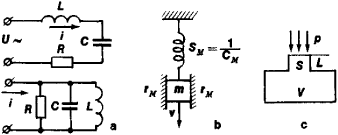# Electroacoustical and Electromechanical Analogies

The following article is from The Great Soviet Encyclopedia (1979). It might be outdated or ideologically biased.

## Electroacoustical and Electromechanical Analogies

(dynamical analogies), analogies for the laws of motion (oscillation) of mechanical oscillatory systems and electric circuits. The principal advantage of such analogies is that they make it possible to use the analytical and design methods of electric oscillatory systems in considering the properties of mechanical and acoustical systems (Figure 1), based on the similarity of the differential equations that describe the state of the systems.Figure 1. Examples of electrical and mechanical analogues: (a) series and parallel isolated electric circuits, (b) mechanical system with one degree of freedom, (c) acoustic resonator

From a comparison of similar equations, Table 1 may be compiled to show the correspondence between electrical, mechanical, and acoustical analogues; depending on whether the equation of a series or a parallel electric circuit is chosen for the comparison, a distinction is made between the first (direct) and second (inverted) systems of analogies. In considering acoustic systems the first system of analogies is more commonly used.

Electroacoustical and electromechanical analogies are especially useful for determining the characteristics of complex mechanical systems with several degrees of freedom, which are very laborious to investigate analytically by solving differential equations. Such systems can be presented in the form of a set of electric networks, and the electric circuit (equivalent circuit) thereby obtained can be analyzed by means of methods used in electrical engineering. The method of analogies is used to design electromechanical and electroacoustical transducers.

### REFERENCES

Furduev, V. V. Elektroakustika. Moscow-Leningrad, 1948.
Olson, H. F. Dinamkheskie analogii. Moscow, 1947. (Translated from English.)
Matauschek, J. Ul’trazvukovaia tekhnika. Moscow, 1962. (Translated from German.)